# Ex 2.1 NCERT Solutions - Polynomials Class 9 Notes | EduRev

## Class 9 : Ex 2.1 NCERT Solutions - Polynomials Class 9 Notes | EduRev

The document Ex 2.1 NCERT Solutions - Polynomials Class 9 Notes | EduRev is a part of the Class 9 Course Class 9 Mathematics by VP Classes.
All you need of Class 9 at this link: Class 9

Ques 1: Which of the following expressions are polynomials in one variable and which are not? State reasons for your answer. (i) 4x2 – 3x + 7 (ii) y2 + 2 (iii)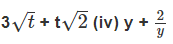(v) x10 + y3 + t50

Ans: (i) 4x2 – 3x + 7
⇒ 4x2 – 3x + 7x0

∵ All the exponents of x are whole numbers.

∴ 4x2 – 3x + 7 is a polynomial in one variable.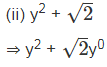∵ All the exponents of y are whole numbers.

∴  y2 +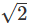is a polynomial in one variable.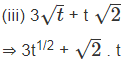∵  1/2 is not a whole number,

∴  3t1/2 +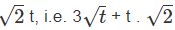is not a polynomial.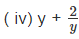ﬁ y + 2 ◊ y–1

∵ (–1) is not a whole number.

∴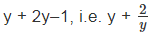is not a polynomial.

(v) x10 + y3 + t50

∵ Exponent of every variable is a whole number,

∴ x10 + y3 + t50 is a polynomial in x, y and t, i.e. in three variables.

Ques 2: Write the co-efficients of x2 in each of the following:

(i) 2 + x2 + x (ii) 2 – x2 + x3 (iii)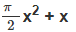(iv)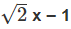Ans: (i) 2 + x2 + x The co-efficient of x2 is 1.

(ii) 2 – x2 + x3 The co-efficient of xis (–1).

(iii)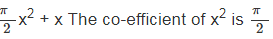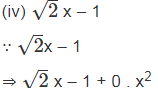∴  The co-efficient of x2 is 0.

Ques 3: Give one example each of a binomial of degree 35, and of a monomial of degree 100.
Ans: (i) A binomial of degree 35 can be: 3x35 – 4

(ii) A monomial of degree 100 can be: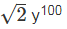Ques 4: Write the degree of each of the following polynomials:
(i) 5x3 + 4x2 + 7x
(ii) 4 – y2
(iii)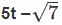(iv) 3

Ans: (i) 5x3 + 4x2 + 7x

∵ The highest exponent of x is 3.

∴ The degree of the polynomial is 3.

(ii) 4 – y2

∵ The highest exponent of y is 2.

∴ The degree of the polynomial is 2.

(iii)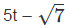∵ The highest exponent of t is 1.

∴ The degree of the polynomial is 1.

( iv) 3 since, 3 = 3x0           [∵ x0 = 1]

∴ The degree of the polynomial 3 is 0.

Ques 5: Classify the following as linear, quadratic and cubic polynomials:

(i) x+ x (ii) x – x3 (iii) y + y2 + 4 (iv) 1 + x ( v ) 3t (vi) r(vii) 7x3
Ans:

(i) x2 + x ∵ The degree of x2 + x is 2.

∴ It is a quadratic polynomial.

(ii) x – x3

∵ The degree of x – x3 is 3.

∴ It is a cubic polynomial.

(iii) y + y+ 4

∵ The degree of y + y2 + 4 is 2.

∴ It is a quadratic polynomial.

(iv) 1 + x

∵ The degree of 1 + x is 1.

∴ It is a linear polynomial.

(v) 3t

∵ The degree of 3t is 1.

∴ It is a linear polynomial.

(vi) r

∵ The degree of r2 is 2.

∴ It is a quadratic polynomial.

(vii) 7x3

∵ The degree of 7xis 3.

∴ It is a cubic polynomial.

Offer running on EduRev: Apply code STAYHOME200 to get INR 200 off on our premium plan EduRev Infinity!

132 docs

,

,

,

,

,

,

,

,

,

,

,

,

,

,

,

,

,

,

,

,

,

;